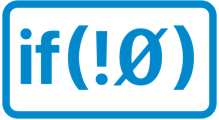# Type of object value in JSON in Xojo

JSON values are variants. Is there a way to tell what type of value they WERE? String, Number, Boolean, etc.?

JSON.Value(“ChVal”) = “SomeText”
JSON.Value(“IntVal”) = 1234
JSON.Value(“BoolVal”) = true

Can I determine what type of value each is after the fact so that if I edit the values, I can make sure they are put back as the same type?

Yes, Variants have a `Type` property that tells you exactly that.

``````dim intVariant as Variant = 1

Select Case intVariant.Type
Case Variant.TypeInteger
...
Case Variant.TypeString
...
End Select
``````
1 Like

Ah! how handy. Thanks!

damn … track record of being first shot to hell1 Like

You can even identify Objects:

``````Select Case myVariant.Type
Case Variant.TypeObject
if (myVariant.ObjectValue IsA Dictionary) then
...
end if
End Select
``````Is there a reverse function like

``````JSON.Value = intVariant.toType(JSON.Value.Type)
``````
``````JSON.Value = intVariant.IntegerValue
``````

But you shouldn’t need to do that. Just assign the Variant.

1 Like

Oh, so you think it’ll retain it’s “type” upon assignment to the JSON? Cool if it does. I’ll give that a try.
Saves a lot of “select cases” and conversions.

Oh, my question wasn’t correct.

It’s going from a string (a ListBox.Cell(row, column)) back to the JSON.Value.

Apropos “hell”. This is something I came across just the other day. Hadn’t seen it for some time…

So I assume I’d have to do:

``````Select Case JSON.Value(Name).Type
Case Variant.TypeInteger
JSON.Value(Name) = Val(Cell(row, column))
Case Variant.TypeString
JSON.Value(Name) = Cell(row, column)
etc
etc
``````

Just assign that cell to your JSON item.

``````JSON.Value = ListBox.Cell(row, column)
``````

Should be all you need to do. Or the other way round:

``````ListBox.Cell(row, column) = JSON.Value

// or, if you want to be more explicit
ListBox.Cell(row, column) = JSON.Value.StringValue
``````

Aren’t all ListBox.Cells Strings rather than Variants?

If I do

``````JSON.Value(Name) = ListBox.Cell(row, column)
``````

That will turn that JSON value into a string, no?

Just confirmed. It will change it to a string.

So Select Case is the solution? No reverse-conversion functions?

Yes, but Variants can take any data type. A Variant doesn’t turn into a datatype when you assign it a value, rather it holds data of that type. As long as we’re talking about basic types a Variant will convert its value to the type of the variable it is assigned to:

``````dim i as Integer = 3
dim v as Variant = i
dim s as String  = v // s = "3"

// or
dim b as Boolean = true
dim v as Variant = b
dim i as Integer = v // i = 1
dim s as String  = v //s = "true"
``````

You’re missing the part about editing the JSON using a ListBox. All ListBox cells are strings. So, I guess I could use a columnTag to keep track of the type of data.

Variant is missing a conversion function that takes one of it’s constants and returns that type.

``````myVariant = Variant.Parse(myStringValue, 3)) ' myVariant is holding a TypeInt64 value
``````

Ah, yes, I didYou could write your own extension method:

``````Sub Parse(Extends myVariant As Variant, newValue As Variant) As Variant
Select Case myVariant.Type
Case Variant.TypeInteger
return newValue.IntegerValue
Case Variant.TypeString
return newValue.StringValue
Case ...
...
else
return newValue // just to be sure you didn't miss a type...
End Select
End Sub
``````

And then

``````myVariant = myVariant.Parse(myStringValue)
``````

Or to make the method more generic:

``````Sub Parse(data As Variant, intoType As Integer) As Variant
Select Case intoType
Case Variant.TypeInteger
return data.IntegerValue
...
End Select
End Sub

...

myVariant = Parse(myStringValue, Variant.TypeInteger)
``````

ah listbox cells ONLY ever contain strings
maybe attach the original value or the type to the celltag so you know what to put it back as ?